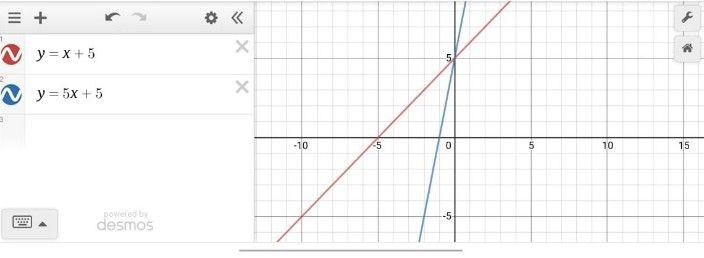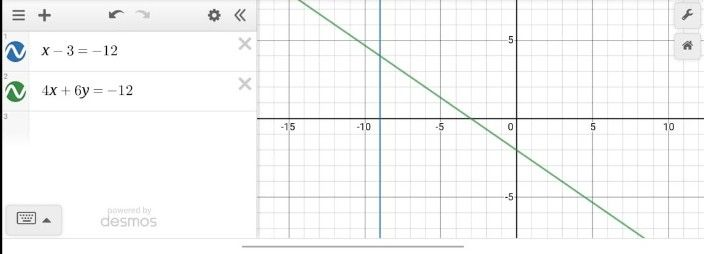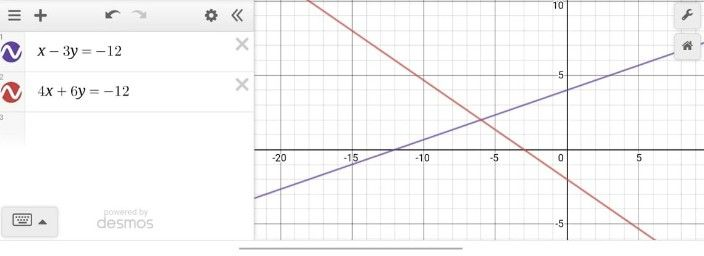Symbol
ProblemLearning Task $3$ Graph the $system$ of equations $1$ $y=x+5$ and $y=5x+5$ $2$ $x-3y=-12$ and $4x+6y=-12$ $3$ $x+2y=-5$ and $-2x+y=3$ $4$ $y=3x-2$ and $y=-x-6$ $5$ $2x-3y=-2$ and $4x+y=24$
Other
Question content
plss help me
SolutionQanda teacher - Muskan12This is for question no. 1Student
teacherQanda teacher - Muskan12
Yes please let me know if you have any queries or issuesStudent
okQanda teacher - Muskan12
actually the above graph equation is wrongStudent
teacher 1 and 2 where is 3 4 5Qanda teacher - Muskan12
so i'm sending you the correct oneStudent
thanksQanda teacher - Muskan12This is the answer for question no 2Student
ok where number 3 and 4.5
my teacherQanda teacher - Muskan12
sending you in 2 minStudent
thanks my teacher ?